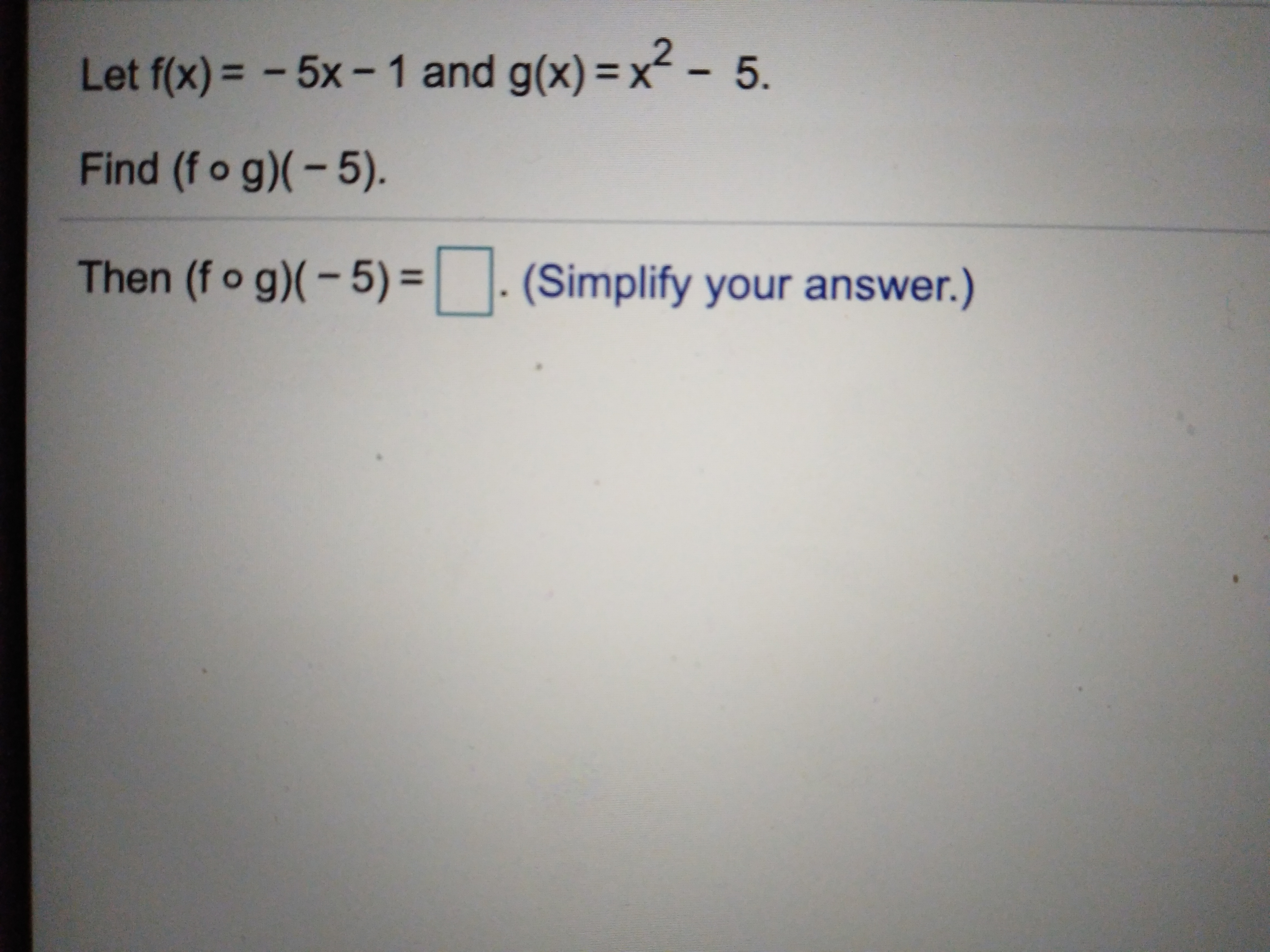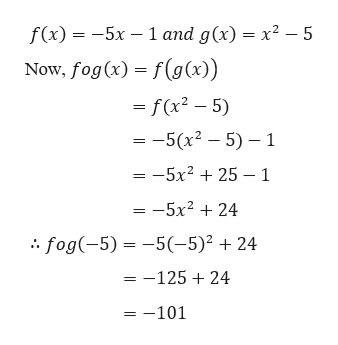# Let f(x)= -5x-1 and g(x)- x2- 5.Find (f o g)-5).Then (fo g)(-5) = | |. (Simplify your answer.)

Question
7 viewshelp_outlineImage TranscriptioncloseLet f(x)= -5x-1 and g(x)- x2- 5. Find (f o g)-5). Then (fo g)(-5) = | |. (Simplify your answer.) fullscreen
check_circle

Step 1

Given,

...help_outlineImage Transcriptionclosef(x)5x 1 and g(x) = x2 - 5 Now, fog(x) f(g(x)) - f(x2 - 5) =-5(x2 5) 1 — -5х2 + 25 ——1 = -5x2 + 24 fog(-5) = -5(-5)2 24 = -125 24 =-101 fullscreen

### Want to see the full answer?

See Solution

#### Want to see this answer and more?

Solutions are written by subject experts who are available 24/7. Questions are typically answered within 1 hour.*

See Solution
*Response times may vary by subject and question.
Tagged in

### Algebra